# Truck

In 7 hours started from town Krnov truck at speed 40 km/h. Passenger car started against it in 8 hours 30 minutes from the city of Jihlava at speed 70 km/h. Distance between this two cities is 225 km. At what time and at what distance from Krnov this two cars meet? Sketch the situation.

Result

t = 10:00 hs =  120 km

### Step-by-step explanation:

225 = 40x + 70(x-1.5)
s = 40x
t = 7+x

225 = 40•x + 70•(x-1.5)
s = 40•x
t = 7+x

110x = 330
s-40x = 0
t-x = 7

s = 120
t = 10
x = 3

Our linear equations calculator calculates it.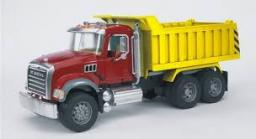Did you find an error or inaccuracy? Feel free to write us. Thank you!Tips to related online calculators
Do you have a linear equation or system of equations and looking for its solution? Or do you have a quadratic equation?
Do you want to convert length units?
Do you want to convert velocity (speed) units?
Do you want to convert time units like minutes to seconds?

## Related math problems and questions:

• Freight and passenger car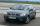The truck starts at 8 pm at 30 km/h. Passenger car starts at 8 pm at 40 km/h. Passenger arrives in the destination city 1 hour and 45 min earlier. What is the distance between the city of departure and destination city?
• Warehouse carsFrom the warehouse started truck at speed 40km/h. After 1hour 30mins started from the same place same direction a car at speed 70 km/h. For how long and at what distance from the warehouse overtake a truck?
• Two citiesCities A and B are 200 km away. At 7 o'clock from city A, the car started at an average speed of 80 km/h, and from B at 45 min later the motorcycle is started at an average speed of 120 km/h. How long will they meet and at what distance from the point A i
• Truck and car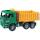At 6h45min, the truck started at a speed of 36 km/h. At 7h30m behind it a passenger car. Find the passenger car's average speed to catch the truck 72 km away from the factory.
• MotionFrom two different locations distant 232 km started against car and bus. The car started at 5:20 with average speed 64 km/h. Bus started at 7:30 with average speed 80 km/h. When they meet? How many kilometers went the bus?
• Two citiesThe distance between cities A and B is 132 km. At 9.00 am, the cyclist started the bike at an average speed of 24 km/h, and at 10.00 am started from the B cyclist at an average speed of 30 km/h. How long and far from A will they both meet?
• Pedestrian and the cyclist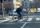Pedestrian and cyclist went against each from the two cities that are distant 40 km. Pedestrian started 30 minutes before the cyclist and walk an average speed of 5 km/h. What speed went cyclist when met after 120 minutes from the start of the pedestrian?
• Passenger car and truckFrom Kutna Hora left at 11:00 clock a truck at 60 km/h. At 12:30 behind him started passenger car at average speed 80 km/h. At what time and how far from Kutna Hora overtake a passenger car truck?
• Cars 2Opava is 360 km distance from Prešov. At 8:00 hour, two cars started against each other from this cities at average speeds 88 km/h and 121 km/h. What hour will it meet and what distance it's travel if the car started from Prešov is faster?
• Two trains meetFrom A started at 7:15 express train at speed 85 km/h to B. From B started passenger train at 8:30 in the direction to A and at speed 55 km/h. The distance A and B are 386 1/4 km. At what time and at what distance from B the two trains meet?
• Two cars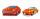From the town A to town B started two cars. The first at 7:00 at average speed 60 km per hour, the second at 10:00 at average speed 100 km per hour. The first car will not stay in B, and on the way back meet the second car at half way from A to B. At what
• Car driver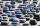The car driver is in town A and is scheduled to be in town B at a specified hour. If it travels at an average speed of 50km/h, it will arrive in city B 30 minutes later. But if he travels at an average speed of 70km/h, he would arrive half an hour earlier
• Cars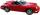Cars started at 7:00 AM. From point A car run at 40 km per hour, from B car run 70 km per hour. Between A and B is a 250 km long road. When and where will meet (measured from point A)?
• Two citiesThe car goes from city A to city B at an average speed of 70 km/h, back at an average speed of 50 km/h. If it goes to B and back at an average speed of 60 km/h, the whole ride would take 8 minutes less. What is the distance between cities A and B?
• Cars speedsDistance from city A to city B is 108 km. Two cars were simultaneously started from both places. The speed of a car coming from city A was 2 km/h higher than the second car. What was the speed of each car if they met in 54 minutes?
• Train from PragueThe first train from Prague started at 8:00 hour at 40 kilometers per hour. Train from Ostrava started at 9:20 at 80 km per hour. How many hours and how far from cities with trains meet if the distance of cities is 400 km.
• Train from BrnoFirst train started from Brno at 8 am at 60 km per hour. Second train started from Opava at 7 o'clock at the speed of 40 kilometers per hour. In how many hours and how far from cities trains meet when the distance of cities is 200 kilometers.